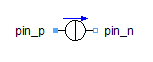Cylinder - MapleSim Help

Cylinder

Cylindrical rigid bodyDescription The Cylinder component models a homogeneous cylindrical rigid body along a given axis with a predefined density. Based on the properties, i.e., axial unit vector, length, radius, and density, the center of mass, total mass, and moments of inertia are calculated for this rigid body.Connections

 Name Description Modelica ID $\mathrm{frame__a}$ Coordinate on one end of the cylinder axis frame_a $\mathrm{frame__b}$ Coordinate on the other end of the cylinder axis frame_b $\mathrm{frame__c}\left[n\right]$ An array of additional frames on the cylinder axis frame_c[n]Parameters

 Name Default Units Description Modelica ID $\mathrm{e__axis}$ $\left[1,0,0\right]$ - Axial unit vector e_axis L $1$ m Cylinder length L $R$ $0.1$ $m$ Cylinder radius R $\mathrm{R__i}$ 0 m Cylinder inner radius R_i Select density User defined - Select a predefined material density selectDensity $\mathrm{ρ}$ 1000 $\frac{\mathrm{kg}}{{m}^{3}}$ Cylinder user-defined material density customDensity Use additional frames false - True means additional frames can be added addFrames $\mathrm{L__add}$ $\left[\frac{L}{2}\right]$ m Each value in this array defines a frame on the cylinder axis w.r.t. frame_a L_add[:] Show visualization true - True means the cylinder geometry is visible in the 3-D playback visualization Transparent false - True means the geometry is transparent in the 3-D playback transparent Color- Cylinder color in the 3-D playback colorEquations The two end frames of the cylinder have the same orientation. The translation vectors  and  w.r.t. frame_a define the frame_b and the center of mass frame, respectively. Cylinder mass is calculated as where the cylinder material density, ρ, can be defined using the "Select density" parameter. This parameter lets the user either enter a value or select among predefined material densities.Figure 1: Different options for the "Select density" property   Assuming the default direction of $\left[1,0,0\right]$ for the cylinder axis, the moments of inertia expressed from the center of mass frame are $\mathrm{I__zz}=\mathrm{I__yy}$ The right-hand side of these equations will interchange if another axial unit vector is specified.ExamplesSwinging T-Shaped Object

Figure 2 shows the layout of a MapleSim model that uses two Cylinder components to simulate a freely swinging T-shaped object. Note how the frame_b of the vertical cylinder (axis = [0,1,0]) is connected to the frame_c of the horizontal cylinder (axis=[1,0,0]) to form the T-shaped object. For the horizontal cylinder, frame_c is located halfway $\left(\mathrm{L__add}=\left[\frac{L}{2}\right]\right)$ between frame_a and frame_b. A snapshot of the 3-D playback is shown in Figure 3.Figure 2: Model layout Figure 3: 3-D playback snapshotSlider-Crank Mechanism In the following example, four Cylinder components are connected with revolute and prismatic joints, as shown in Figure 5, to model a slider-crank mechanism. Using Cylinder components facilitates the modeling by automatically calculating mass and moments of inertia and also results in realistic visualization in the 3-D playback window, as shown in Figure 6.Figure 5: Model layoutFigure 6: 3-D playback snapshot